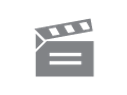Media not available in the Digital Archive
Description
This programme shows a proof of the fundamental theorem of calculus and one way it can be used.
Module code and title: M101, Mathematics: a foundation course M101; 13; 1983 1983 00:24:30 + Show more... Pip Surgey Mike Simpson; Anne Walton BBC Open University Area of circle; Calculus; Computer; Cosine; Fundamental theorem; Integration; Rectangles The formula Pi r2 for the area of a circle is so familiar that most people quote it without thinking about where it came from; it's a result that is taken for granted. Equally taken for granted is the idea that integration and differentiation are "reverse" activities. Few students ever question this result, yet it is the basis of one of the most important theorems in mathematics, the Fundamental Theorem of Calculus. In this programme, which is associated with unit 3 of Block III (the Calculus block), students are shown a proof of the Fundamental Theorem, and how it can be applied to prove the formula for the area of a circle. Initially students are posed a question; how would they prove that the area of a circle can be found using Pi r2? Using a quarter circle for simplicity, the programme briefly recaps the concept of integration as the limit of the upper and lower sums of rectangles. As the cosine function is easier to handle than that for the circle the programme then moves on to investigate integrals of the cosine function by summation, initially to reinforce the idea of the limit of a sum, and then to show the relationship between area under a curve and integral. All the intervals used have the left hand limit as O, and this leads-on to the definition of the function (...). HOU4527 FOUM194X 2469 no